# RD Sharma Solutions Class 9 Herons Formula Exercise 12.2

RD Sharma Solutions for Class 9 Maths are given here for Chapter 12 exercise 12.2. In this exercise, students will deal with the various applications of Heron’s formula. For example, if a landowner needs to find out the area of his land which is in the shape of a quadrilateral. He needs to divide the quadrilateral into triangular parts and use the heron’s formula for the area of a triangular part. Learn to apply heron’s formula easily by understanding the practice problems that are solved in RD Sharma class 9 chapter 12 solutions given here

## Download PDF of RD Sharma Solutions for Class 9 Maths Chapter 12 Heron’s Formula Exercise 12.2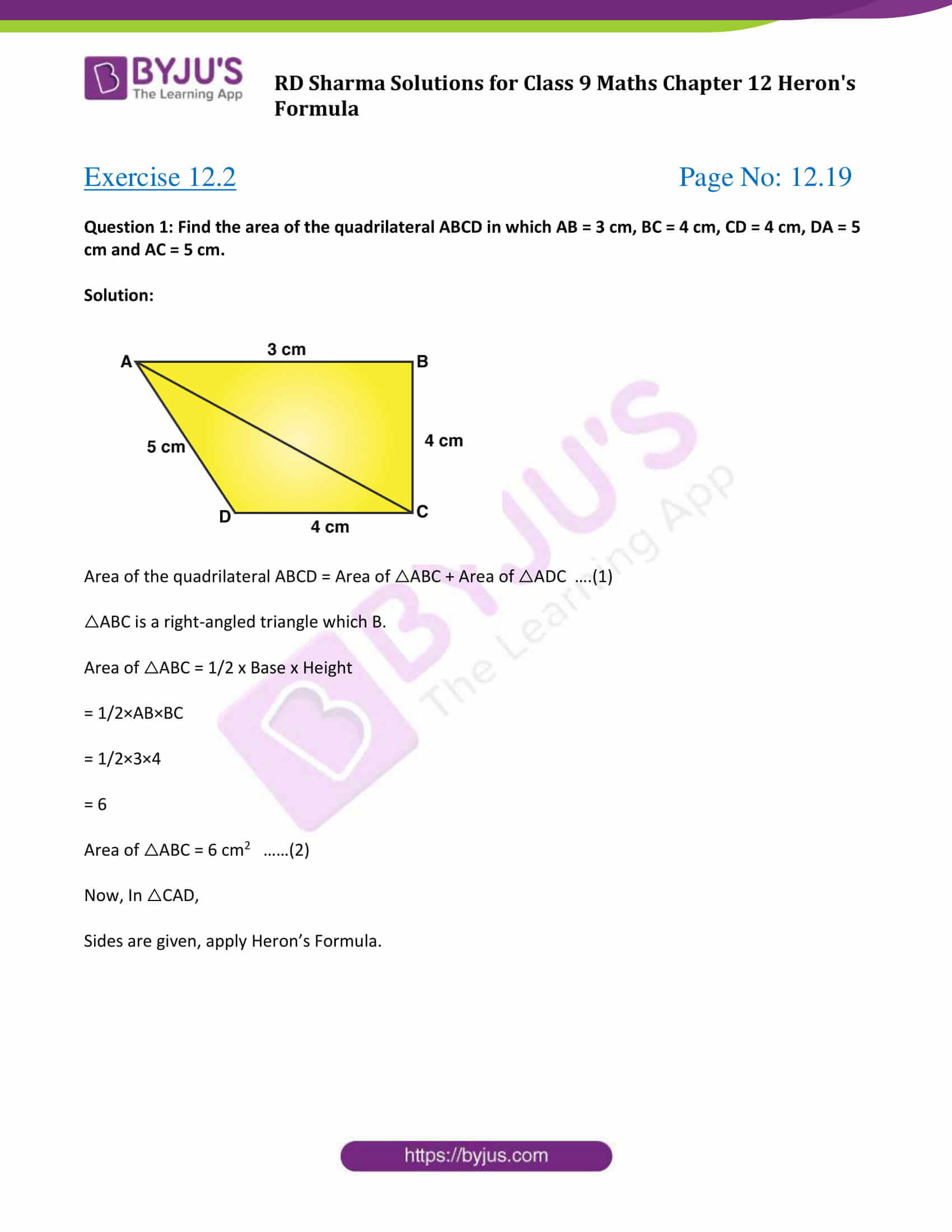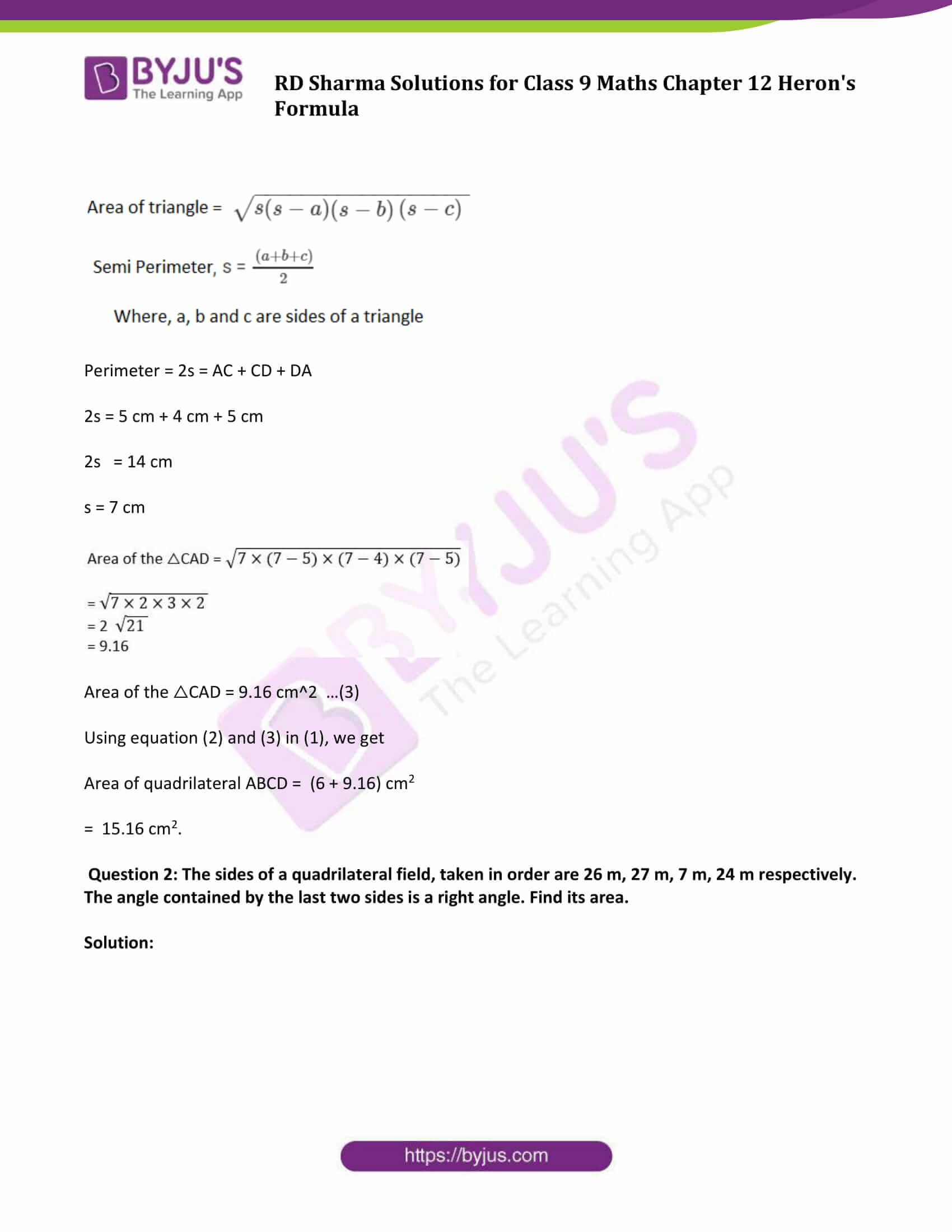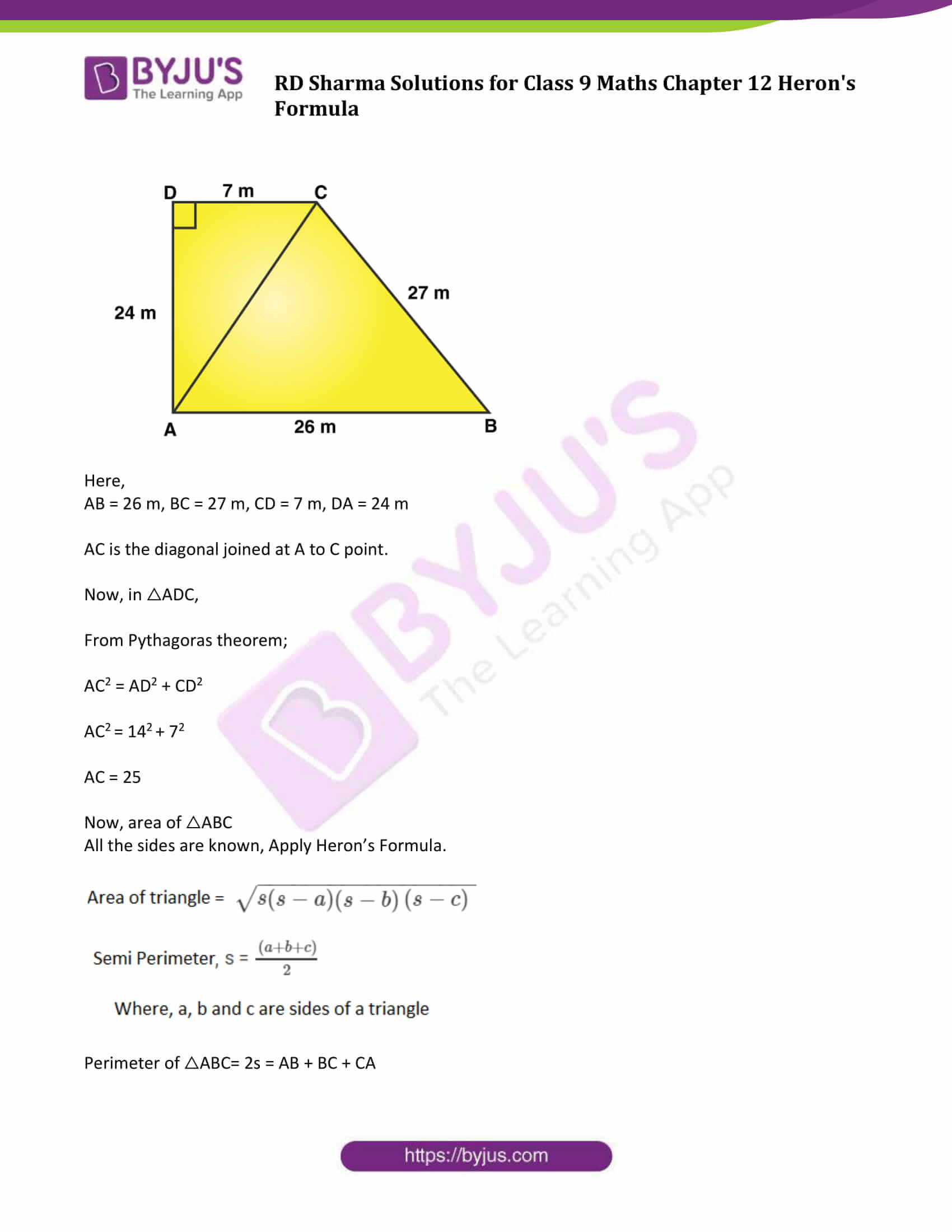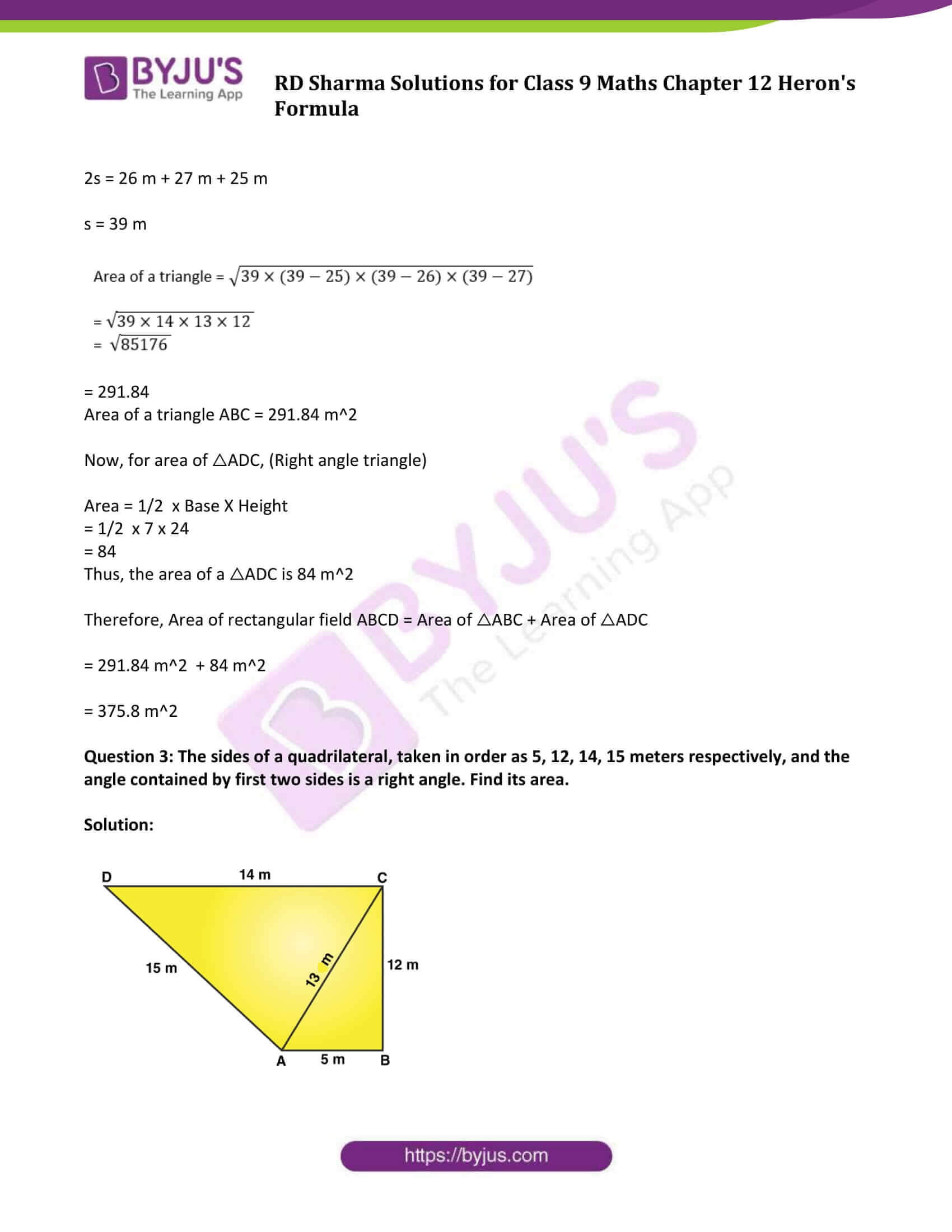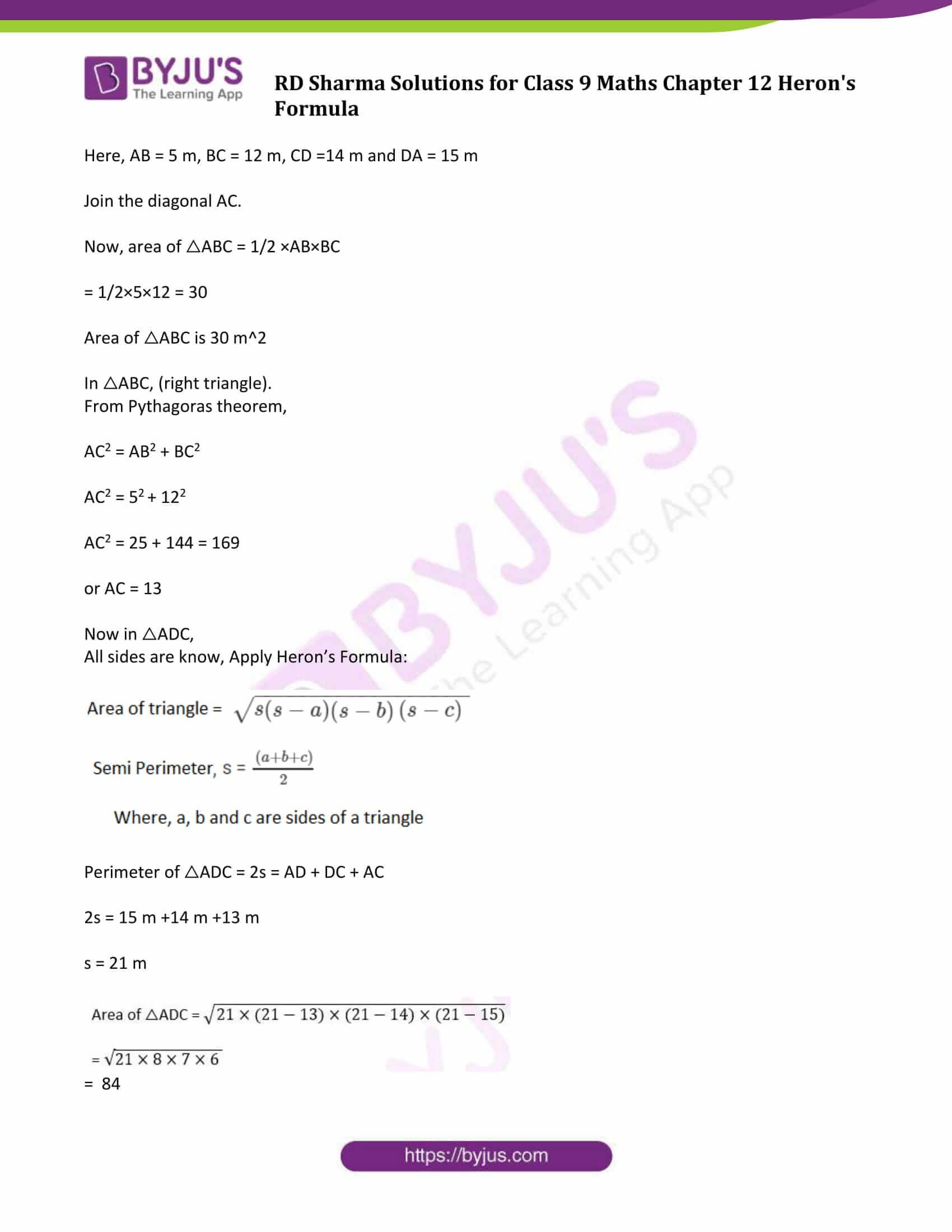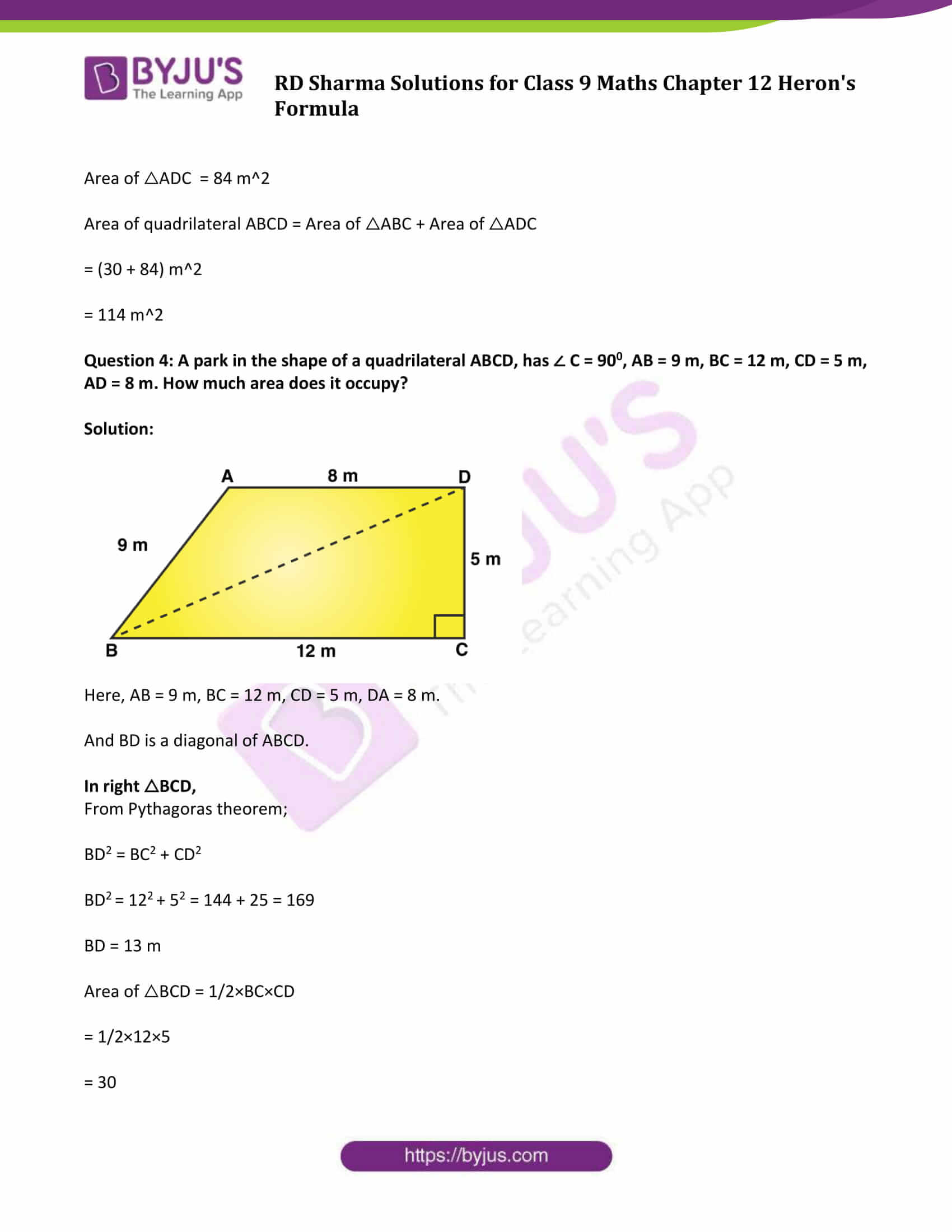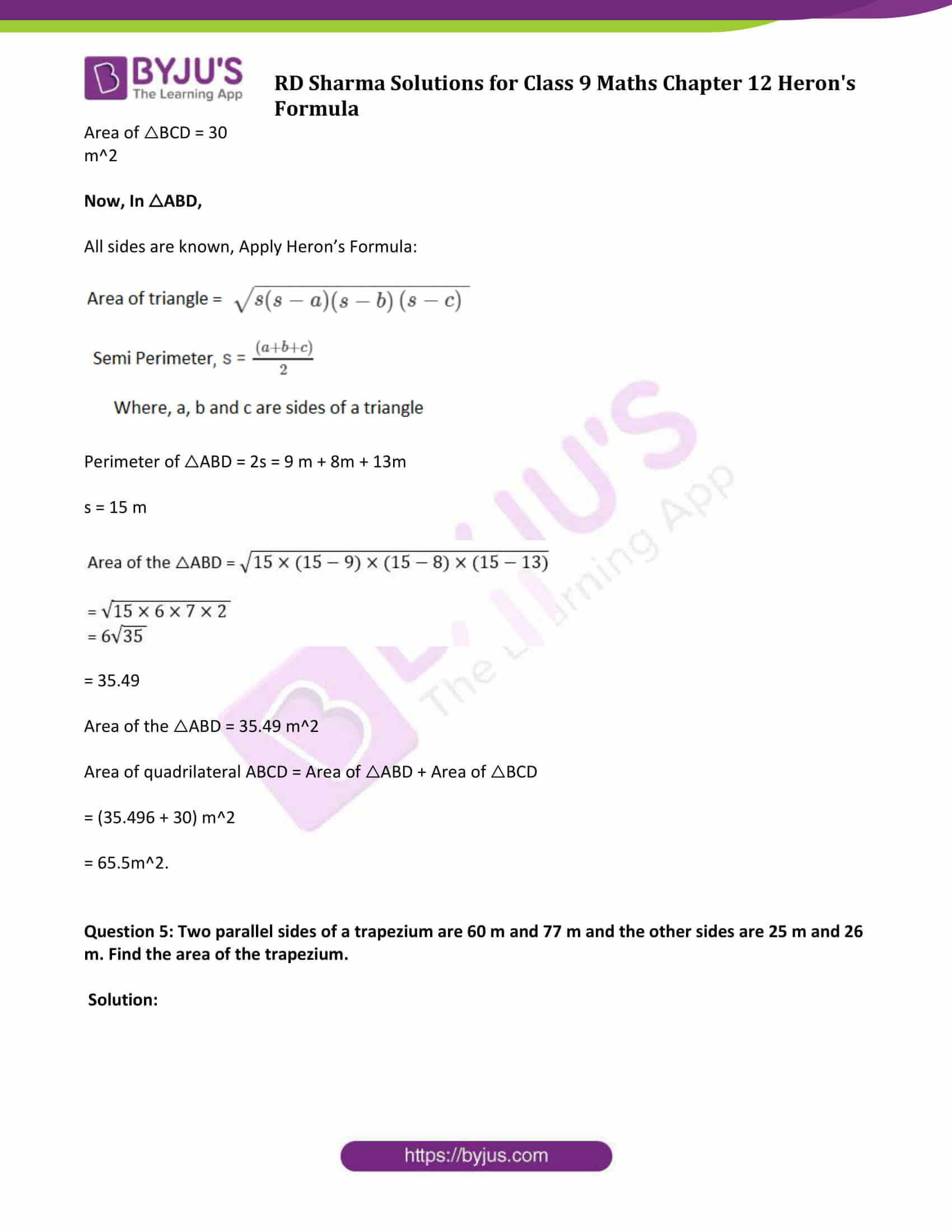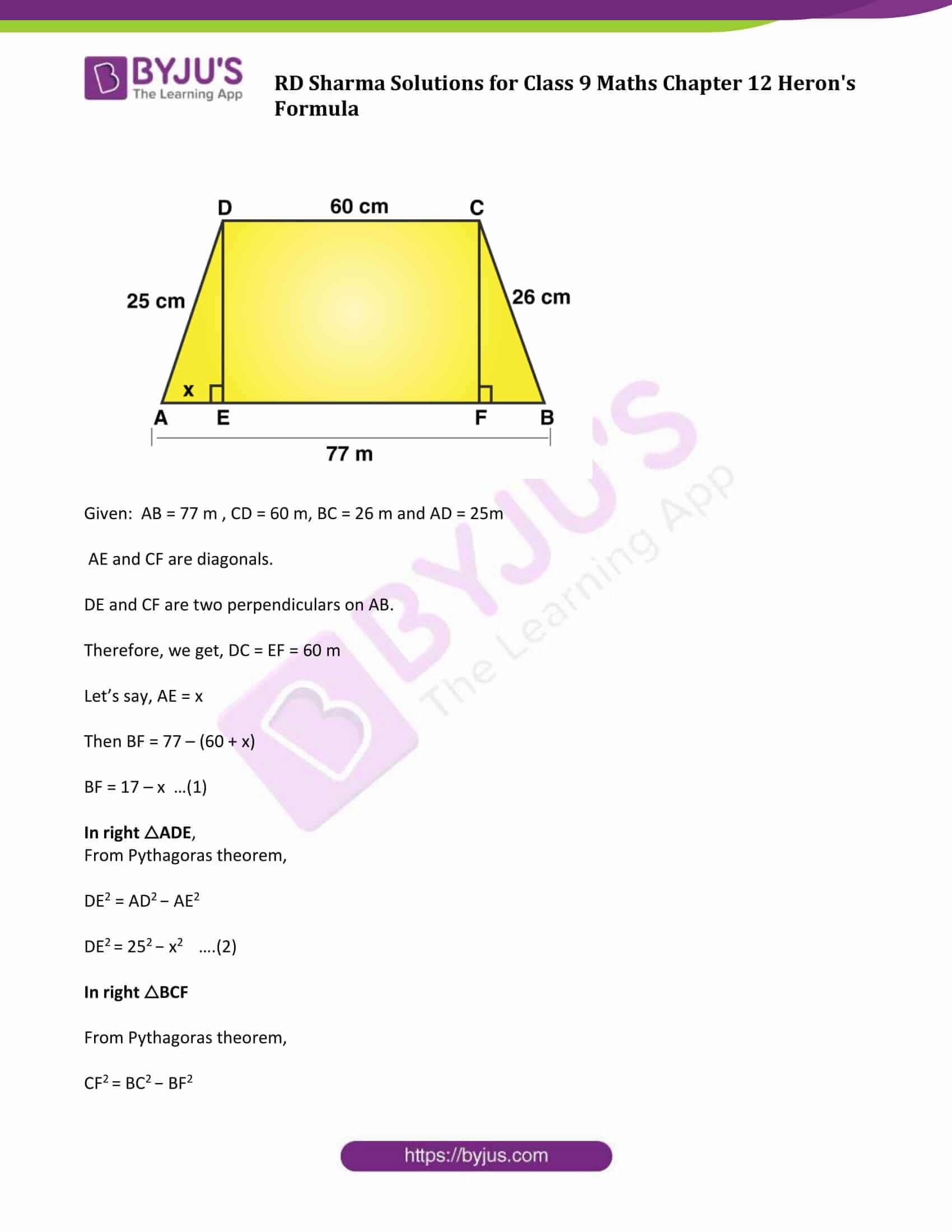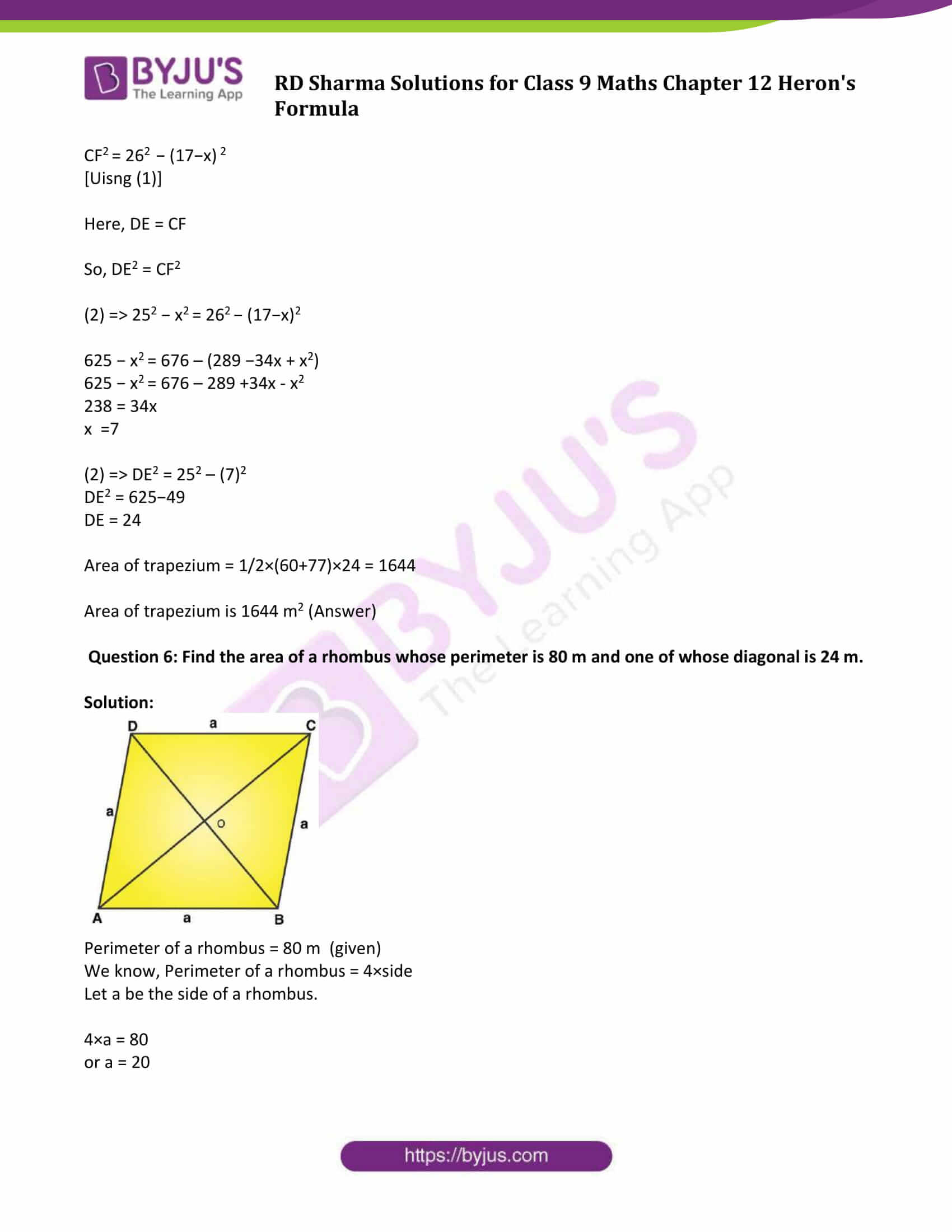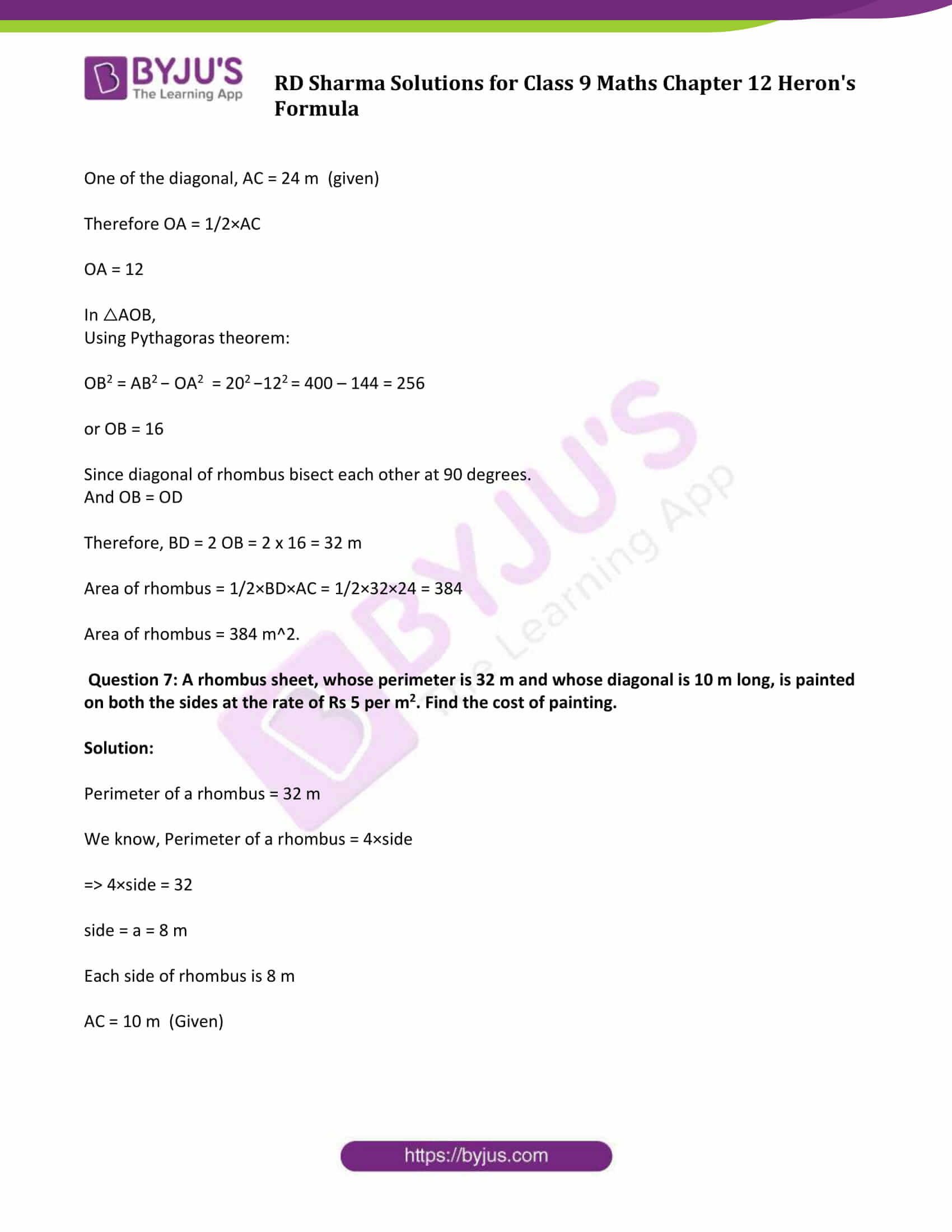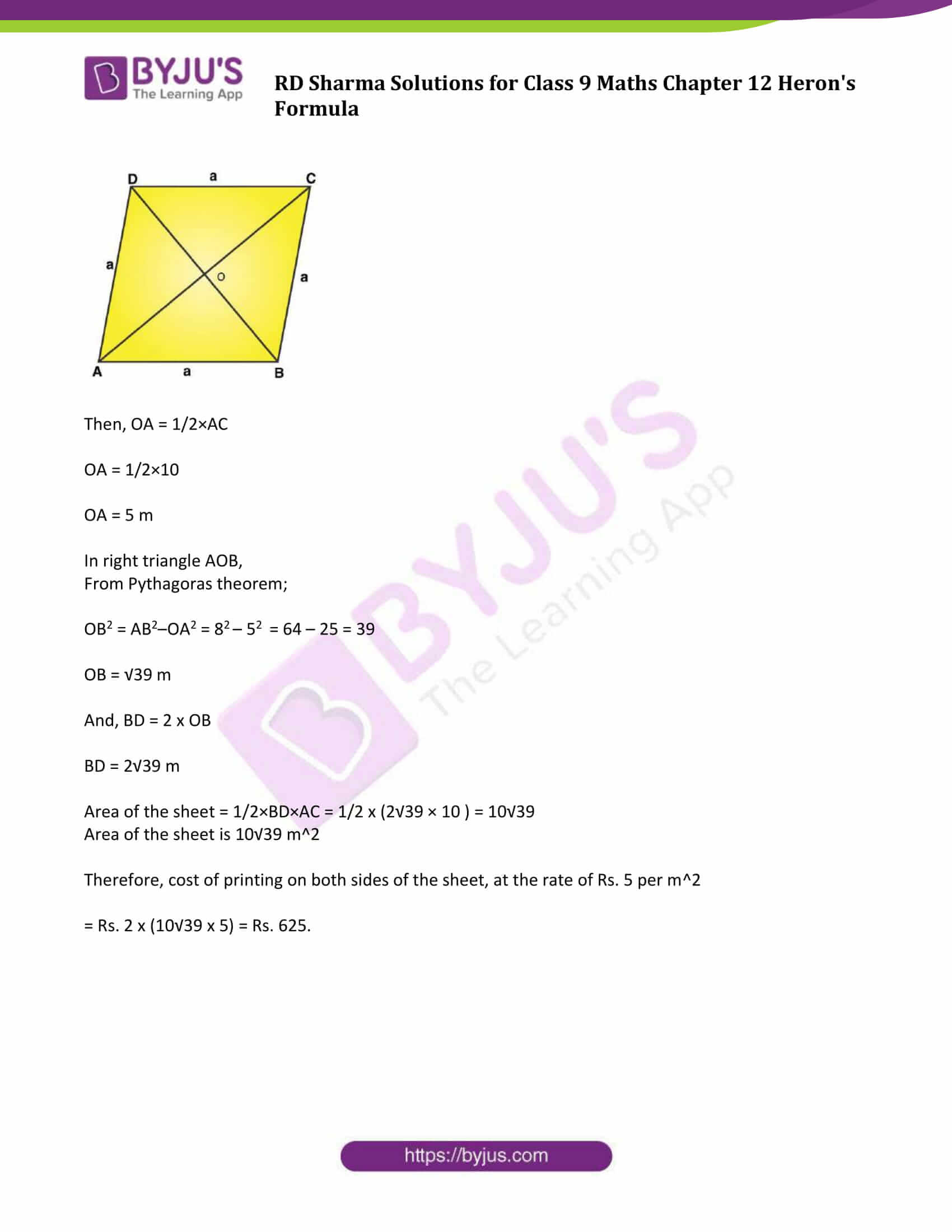### Access Answers to Maths RD Sharma Class 9 Chapter 12 Heron’s Formula Exercise 12.2 Page number 12.19

Question 1: Find the area of the quadrilateral ABCD in which AB = 3 cm, BC = 4 cm, CD = 4 cm, DA = 5 cm and AC = 5 cm.

Solution: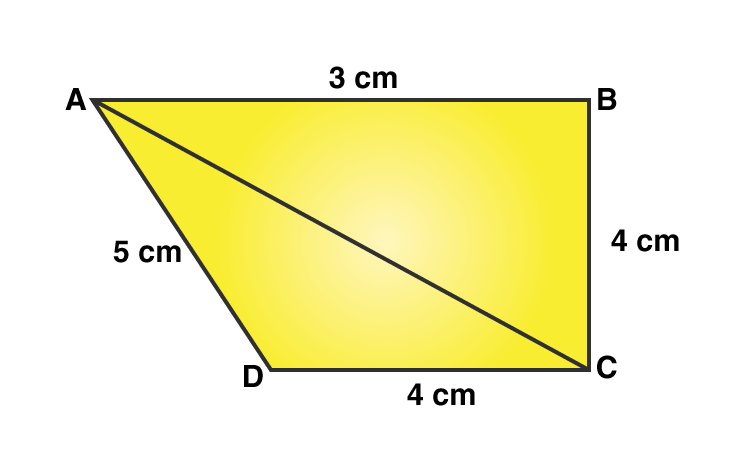Area of the quadrilateral ABCD = Area of △ABC + Area of △ADC ….(1)

△ABC is a right-angled triangle which B.

Area of △ABC = 1/2 x Base x Height

= 1/2×AB×BC

= 1/2×3×4

= 6

Area of △ABC = 6 cm2 ……(2)

Sides are given, apply Heron’s Formula.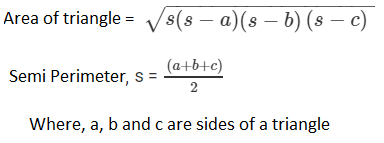Perimeter = 2s = AC + CD + DA

2s = 5 cm + 4 cm + 5 cm

2s = 14 cm

s = 7 cm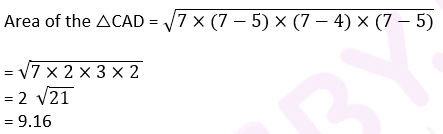Area of the △CAD = 9.16 cm2 …(3)

Using equation (2) and (3) in (1), we get

Area of quadrilateral ABCD = (6 + 9.16) cm2

= 15.16 cm2.

Question 2: The sides of a quadrilateral field, taken in order are 26 m, 27 m, 7 m, 24 m respectively. The angle contained by the last two sides is a right angle. Find its area.

Solution: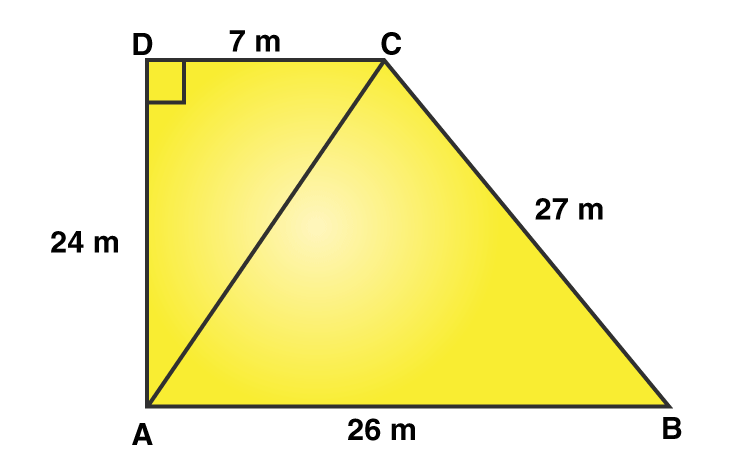Here,

AB = 26 m, BC = 27 m, CD = 7 m, DA = 24 m

AC is the diagonal joined at A to C point.

From Pythagoras theorem;

AC2 = 142 + 72

AC = 25

Now, area of △ABC

All the sides are known, Apply Heron’s Formula.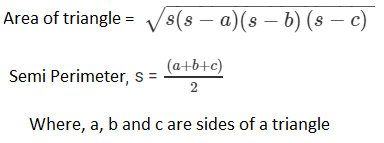Perimeter of △ABC= 2s = AB + BC + CA

2s = 26 m + 27 m + 25 m

s = 39 m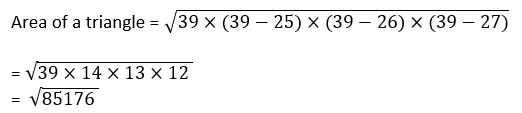= 291.84

Area of a triangle ABC = 291.84 m2

Now, for area of △ADC, (Right angle triangle)

Area = 1/2 x Base X Height

= 1/2 x 7 x 24

= 84

Thus, the area of a △ADC is 84 m2

Therefore, Area of rectangular field ABCD = Area of △ABC + Area of △ADC

= 291.84 m2 + 84 m2

= 375.8 m2

Question 3: The sides of a quadrilateral, taken in order as 5, 12, 14, 15 meters respectively, and the angle contained by first two sides is a right angle. Find its area.

Solution: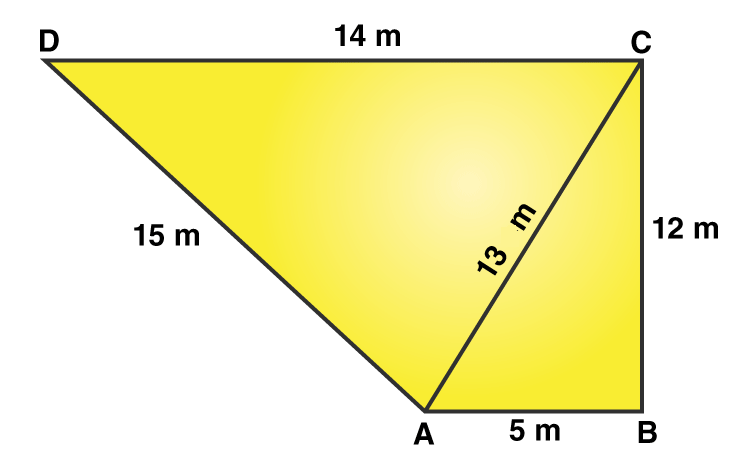Here, AB = 5 m, BC = 12 m, CD =14 m and DA = 15 m

Join the diagonal AC.

Now, area of △ABC = 1/2 ×AB×BC

= 1/2×5×12 = 30

Area of △ABC is 30 m2

In △ABC, (right triangle).

From Pythagoras theorem,

AC2 = AB2 + BC2

AC2 = 52 + 122

AC2 = 25 + 144 = 169

or AC = 13

All sides are know, Apply Heron’s Formula:2s = 15 m +14 m +13 m

s = 21 m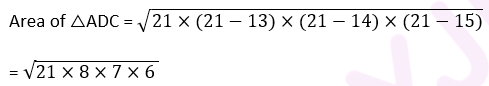= 84

Area of △ADC = 84 m2

Area of quadrilateral ABCD = Area of △ABC + Area of △ADC

= (30 + 84) m2

= 114 m2

Question 4: A park in the shape of a quadrilateral ABCD, has ∠ C = 900, AB = 9 m, BC = 12 m, CD = 5 m, AD = 8 m. How much area does it occupy?

Solution: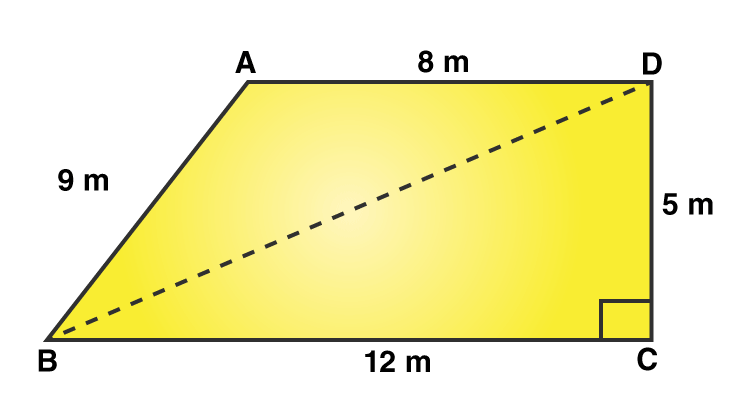Here, AB = 9 m, BC = 12 m, CD = 5 m, DA = 8 m.

And BD is a diagonal of ABCD.

In right △BCD,

From Pythagoras theorem;

BD2 = BC2 + CD2

BD2 = 122 + 52 = 144 + 25 = 169

BD = 13 m

Area of △BCD = 1/2×BC×CD

= 1/2×12×5

= 30

Area of △BCD = 30 m2

Now, In △ABD,

All sides are known, Apply Heron’s Formula:Perimeter of △ABD = 2s = 9 m + 8m + 13m

s = 15 m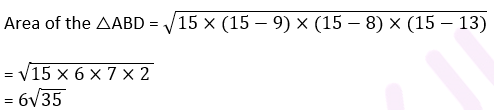= 35.49

Area of the △ABD = 35.49 m2

Area of quadrilateral ABCD = Area of △ABD + Area of △BCD

= (35.496 + 30) m2

= 65.5m2.

Question 5: Two parallel sides of a trapezium are 60 m and 77 m and the other sides are 25 m and 26 m. Find the area of the trapezium.

Solution: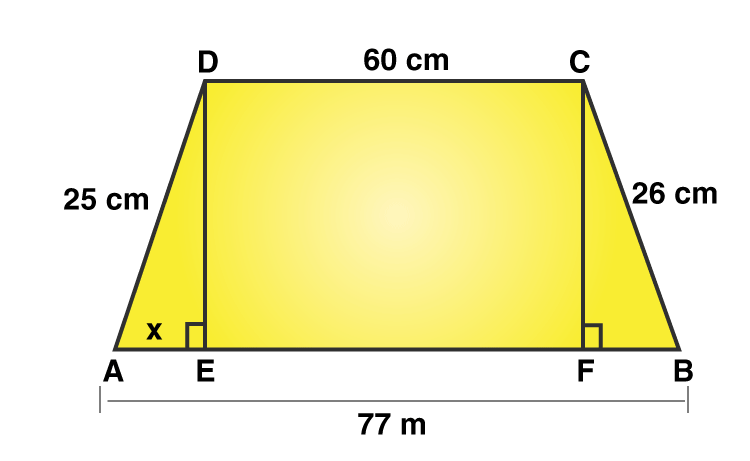Given: AB = 77 m , CD = 60 m, BC = 26 m and AD = 25m

AE and CF are diagonals.

DE and CF are two perpendiculars on AB.

Therefore, we get, DC = EF = 60 m

Let’s say, AE = x

Then BF = 77 – (60 + x)

BF = 17 – x …(1)

From Pythagoras theorem,

DE2 = 252 − x2 ….(2)

In right △BCF

From Pythagoras theorem,

CF2 = BC2 − BF2

CF2 = 262 − (17−x) 2

[Uisng (1)]

Here, DE = CF

So, DE2 = CF2

(2) ⇒ 252 − x2 = 262 − (17−x)2

625 − x2 = 676 – (289 −34x + x2)

625 − x2 = 676 – 289 +34x – x2

238 = 34x

x =7

(2) ⇒ DE2 = 252 – (7)2

DE2 = 625−49

DE = 24

Area of trapezium = 1/2×(60+77)×24 = 1644

Area of trapezium is 1644 m2 (Answer)

Question 6: Find the area of a rhombus whose perimeter is 80 m and one of whose diagonal is 24 m.

Solution: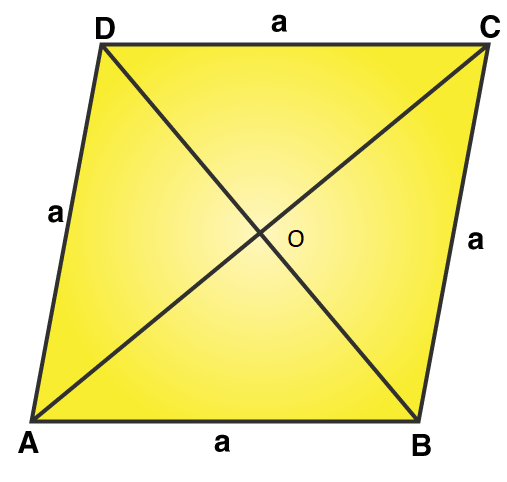Perimeter of a rhombus = 80 m (given)

We know, Perimeter of a rhombus = 4×side

Let a be the side of a rhombus.

4×a = 80

or a = 20

One of the diagonal, AC = 24 m (given)

Therefore OA = 1/2×AC

OA = 12

In △AOB,

Using Pythagoras theorem:

OB2 = AB2 − OA2 = 202 −122 = 400 – 144 = 256

or OB = 16

Since diagonal of rhombus bisect each other at 90 degrees.

And OB = OD

Therefore, BD = 2 OB = 2 x 16 = 32 m

Area of rhombus = 1/2×BD×AC = 1/2×32×24 = 384

Area of rhombus = 384 m2.

Question 7: A rhombus sheet, whose perimeter is 32 m and whose diagonal is 10 m long, is painted on both the sides at the rate of Rs 5 per m2. Find the cost of painting.

Solution:

Perimeter of a rhombus = 32 m

We know, Perimeter of a rhombus = 4×side

⇒ 4×side = 32

side = a = 8 m

Each side of rhombus is 8 m

AC = 10 m (Given)Then, OA = 1/2×AC

OA = 1/2×10

OA = 5 m

In right triangle AOB,

From Pythagoras theorem;

OB2 = AB2–OA2 = 82 – 52 = 64 – 25 = 39

OB = √39 m

And, BD = 2 x OB

BD = 2√39 m

Area of the sheet = 1/2×BD×AC = 1/2 x (2√39 × 10 ) = 10√39

Area of the sheet is 10√39 m2

Therefore, cost of printing on both sides of the sheet, at the rate of Rs. 5 per m2

= Rs. 2 x (10√39 x 5) = Rs. 625.

## RD Sharma Solutions for Class 9 Maths Chapter 12 Exercise 12.2

RD Sharma Solutions Class 9 Maths Chapter 12 Heron’s Formula Exercise 12.2 is based on applications of heron’s formula. All questions enlisted under this exercise are solved by subject experts to serve students better. Find detailed RD Sharma solutions for class 9 Maths here and clear all your doubts.